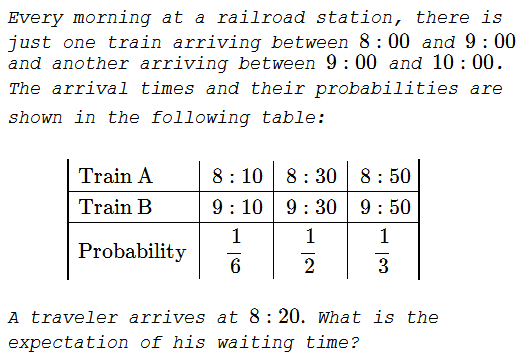# Waiting for a Train

### Problem### Solution

There is the probability of $\displaystyle \frac{1}{6}$ of the traveller missing Train A by $10$ minutes. The distribution of the waiting times is shown in the following table:

$\displaystyle \begin{array}{|l|c|c|c|} \text{Time in min} & 10 & 30 & 50 & 70 & 90\\ \hline \text{Probability} & \displaystyle \frac{1}{2} & \displaystyle \frac{1}{3} & \displaystyle \frac{1}{6}\cdot\frac{1}{6}& \displaystyle \frac{1}{6}\cdot\frac{1}{2}&\displaystyle \frac{1}{6}\cdot\frac{1}{3} \end{array}$

making the expectation:

$\displaystyle 10\cdot\frac{1}{2}+30\cdot\frac{1}{3}+50\cdot\frac{1}{36}+70\cdot\frac{1}{12}+90\cdot\frac{1}{18}=\frac{980}{36}\approx 27\text{ min}.$

### Remark

A curious thing about this problem is that, assuming that the traveler comes a little earlier so as not to miss train A, say at $8:05,$ then the expected waiting time will grow:

$\displaystyle 5\cdot\frac{1}{6}+25\cdot\frac{1}{2}+45\cdot\frac{1}{3}\approx 28.$

### Acknowledgment

This is a problem from a 2009 Chinese Olympiad I found in a book Mathematical Olympiad in China (2009-2010): Problems and Solutions by Xiong Bin and Lee Peng Yee(World Scientific, 2013, 22-23).